Goseeko blog# What is numerical integration?

## Overview (numerical integration)

Numerical integration is the method to calculate the approximate value of the integral by using numerical techniques.

There are various useful and interesting methods for numerical integration such as trapezoidal rule, Simpson’s rules, Gauss’s, Newton-Leibnitz rules etc.

Generally, we use fundamental theorem of calculus to find the solution for definite integrals.

Sometime integration becomes too hard to evaluate therefore, numerical methods are used to find the approximated value of the integral.

## Numerical Integration

Numerical integration is a process of evaluating or obtaining a definite integral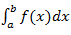from a set of numerical values of the integrand f(x).

In case of function of single variable, we call this as quadrature.

## Trapezoidal Method

Suppose, the interval [a,b] be divided into n equal intervals such that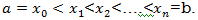Here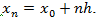To find the value ofSetting n=1, we get

Or

I = ½ (h) [(sum of the first and last ordinates) + 2( sum of all intermediate ordinates)]

The above is the Trapezoidal rule.

Note: In this method second and higher difference has to be neglected and so that f(x) is a polynomial of degree 1.

Geometrical Significance: The curve y=f(x), we replace by n straight lines with the points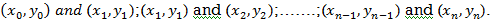The area bounded by the curve y = f(x), the ordinates x=xo and x=xn the x axis is approximately equivalent to the sum of the area of the n trapeziums obtained.

Example: Solve using trapezoidal rule with five ordinates

Sol:

Here, we have

Now, we construct the data table:

I = ½ (h) [(sum of the first and last ordinates) + 2( sum of all intermediate ordinates)]

Simpson’s rules are very useful in numerical integration to evaluate such integrals.

Here we will understand the concept of Simpson’s rule and evaluate integrals by using numerical technique of integration.

We find more accurate value of the integration by using Simpson’s rule than other methods

Therefore we will study about Simpson’s one-third rule and Simpson’s three-eight rules.

But in order to get these two formulas, we should have to know about the general quarature formula-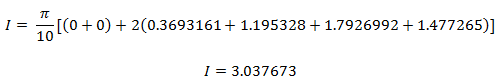## Simpson’s rule

Here we will understand the concept of Simpson’s rule and evaluate integrals by using numerical techniques of integration.

In this paragraph, we are going to discuss two very important methods, Simpson’s one-third rule and Simpson’s three-eight rules.

The general quadrature formula is gives as,

We get Simpson’s one-third and three-eighth formulas by putting n = 2 and n = 3 respectively in the general quadrature formula.

## Simpson’s one-third rule

Put n = 2 in general quadrature formula-

We get-

Note- the given interval of integration has to be divided into an even number of sub-intervals.

## Simpson’s three-eighth rule

Put n = 3 in general quadrature formula-

We get-

Note- the given interval of integration has to be divided into sub-intervals whose number n is a multiple of 3.

## Solved examples of numerical integration

Example: Calculate the value the following integral by using the Simpson’s rules

Solution-

First of all we will divide the interval into six part, where width (h) = 1, the value of f(x) will be

Now we will use Simpson’s 1/3rd rule-

Therefore we get

And now

Now using Simpson’s 3/8th rule-

Example: Evaluate the approximated value of the following integral by using Simpson’1/3rd rule.

Solution-

The table of the values, we get

Now using Simpson’s 1/3rd rule-

We get-

Interested in learning about similar topics? Here are a few hand-picked blogs for you!